# Femtoscopy Theory Scott Pratt Michigan State University Scott

• Slides: 30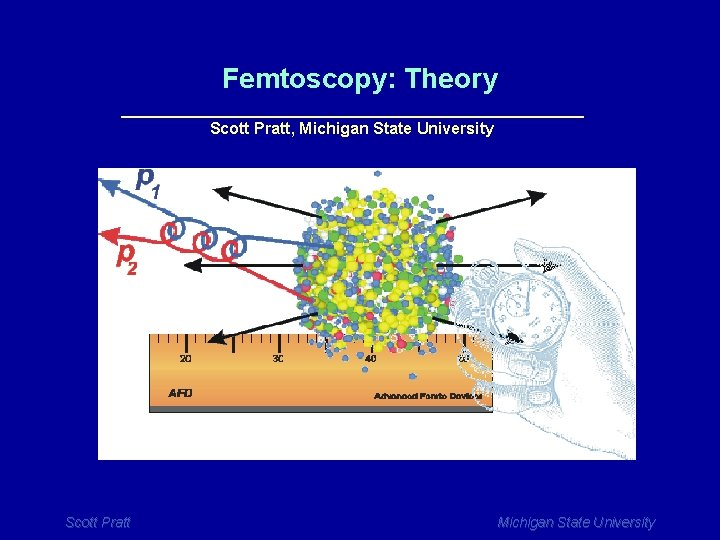Femtoscopy: Theory __________________________ Scott Pratt, Michigan State University Scott Pratt Michigan State University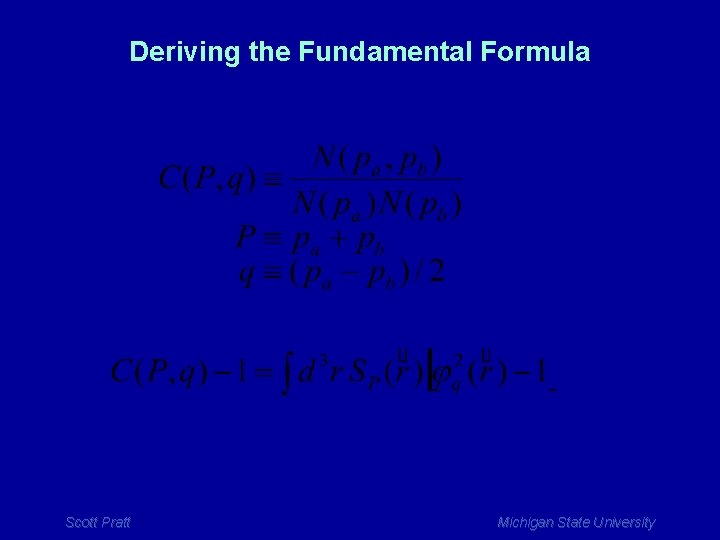Deriving the Fundamental Formula Scott Pratt Michigan State UniversityDeriving the Fundamental Formula Step 1: Define the source function Scott Pratt Michigan State University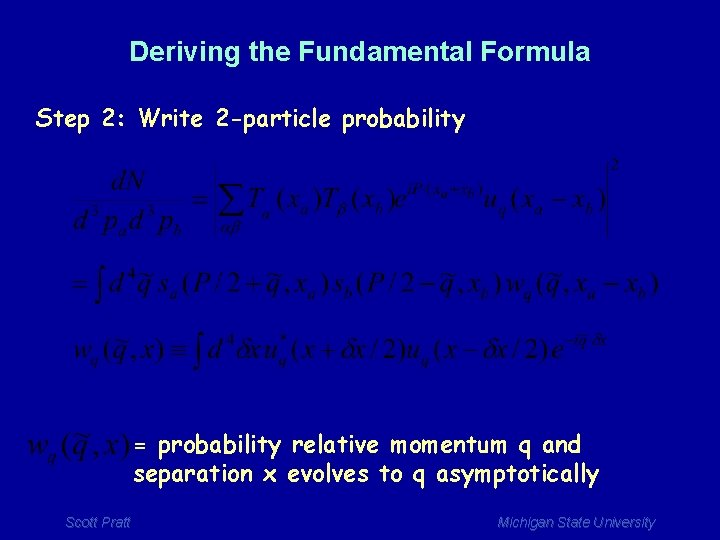Deriving the Fundamental Formula Step 2: Write 2 -particle probability = probability relative momentum q and separation x evolves to q asymptotically Scott Pratt Michigan State University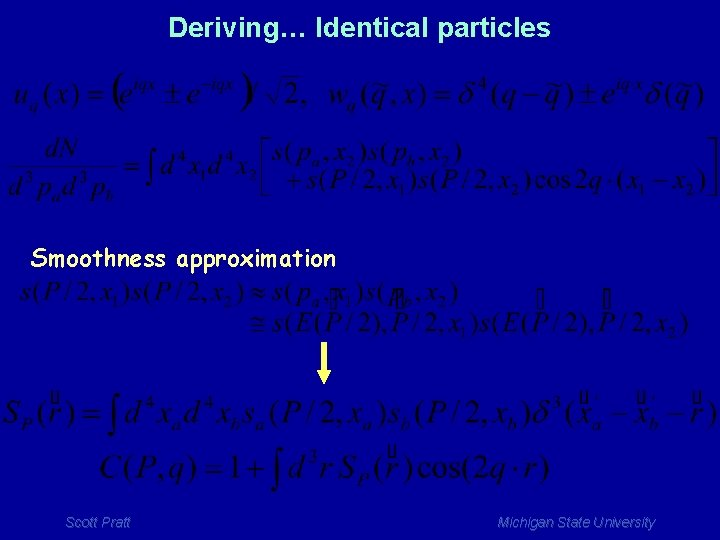Deriving… Identical particles Smoothness approximation Scott Pratt Michigan State UniversityWith final-state interactions Approximate (in frame of pair), Smoothness approximation Scott Pratt Michigan State University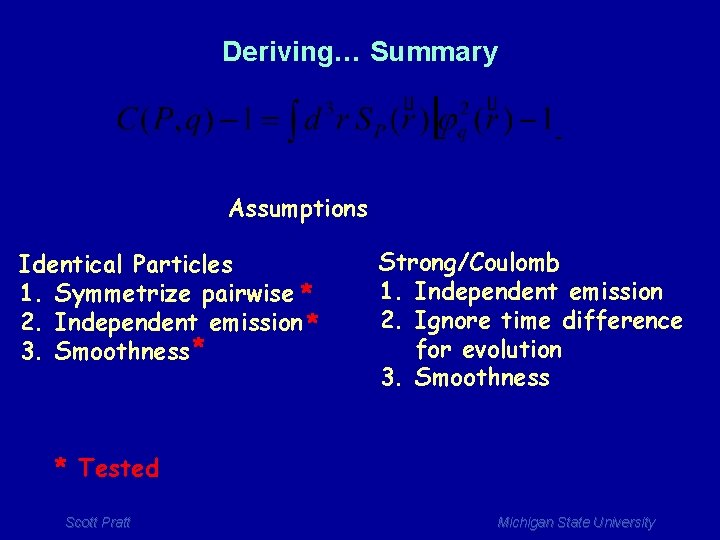Deriving… Summary Assumptions Identical Particles 1. Symmetrize pairwise * 2. Independent emission * 3. Smoothness * Strong/Coulomb 1. Independent emission 2. Ignore time difference for evolution 3. Smoothness * Tested Scott Pratt Michigan State University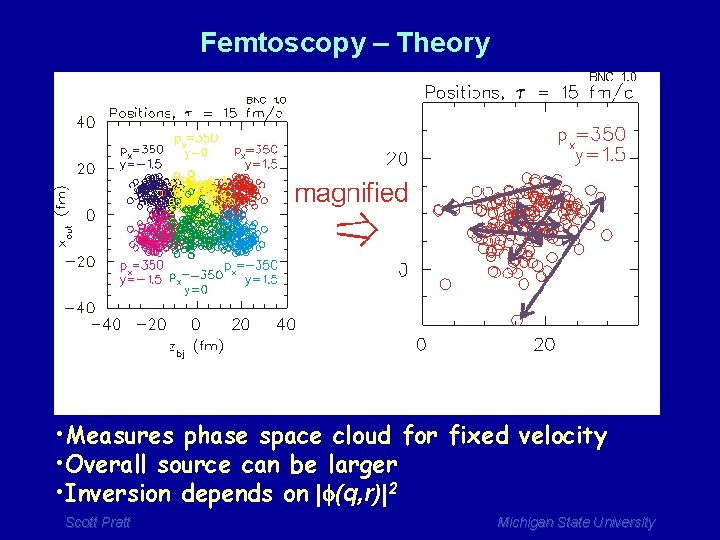Femtoscopy – Theory • Measures phase space cloud for fixed velocity • Overall source can be larger • Inversion depends on |f(q, r)|2 Scott Pratt Michigan State University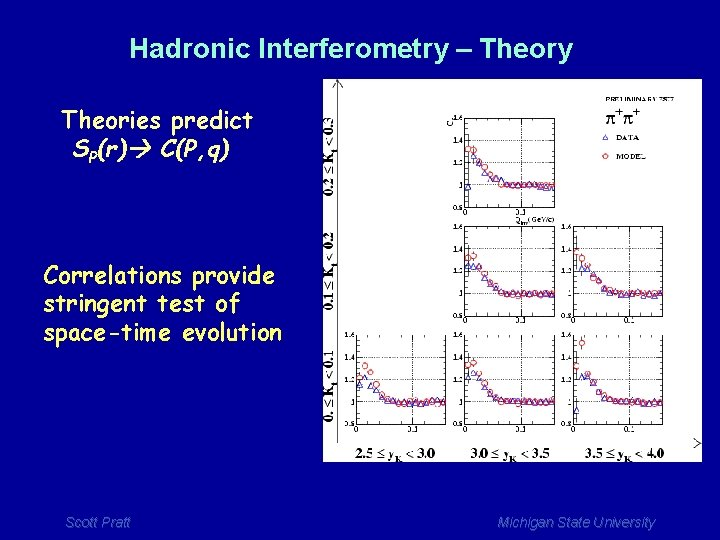Hadronic Interferometry – Theory Theories predict SP(r) C(P, q) Correlations provide stringent test of space-time evolution Scott Pratt Michigan State UniversityUsing Identical Particles • Examples: pp, KK, … • Easy to invert • 3 -dimensional information • Rout, Rside, Rlong are functions of P Scott Pratt Michigan State University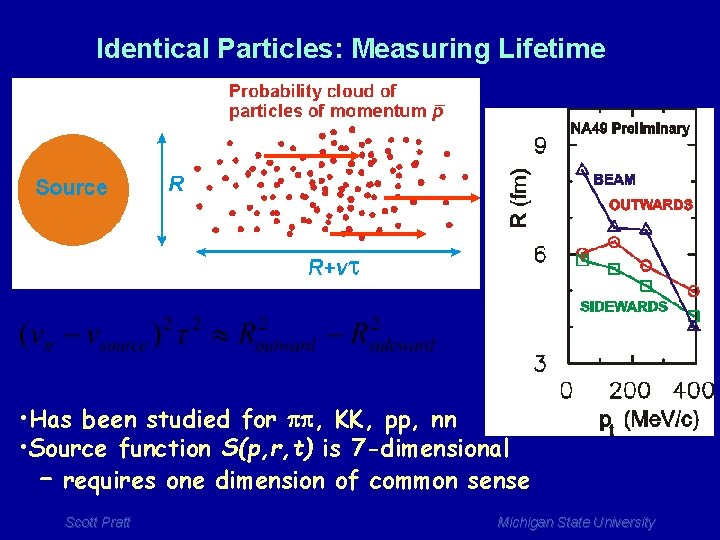Identical Particles: Measuring Lifetime • Has been studied for pp, KK, pp, nn • Source function S(p, r, t) is 7 -dimensional – requires one dimension of common sense Scott Pratt Michigan State University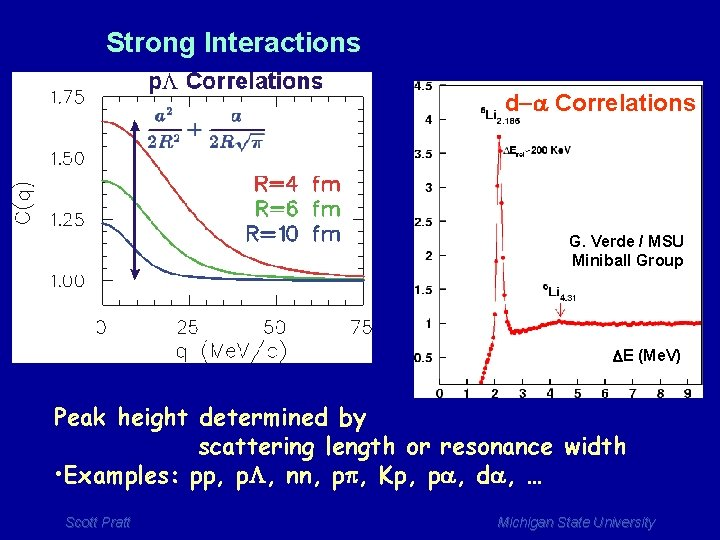Strong Interactions d-a Correlations G. Verde / MSU Miniball Group DE (Me. V) Peak height determined by scattering length or resonance width • Examples: pp, p. L, nn, pp, Kp, pa, da, … Scott Pratt Michigan State UniversityCoulomb Interactions • Can be calculated classically for larger fragments Kim et al. , PRC 45 p. 387 (92) Scott Pratt Michigan State UniversityS. Panitkin and D. Brown, PRC 61 021901 (2000) Proton-proton Correlations Deconvoluting C(q) provides detailed source shape Scott Pratt Michigan State University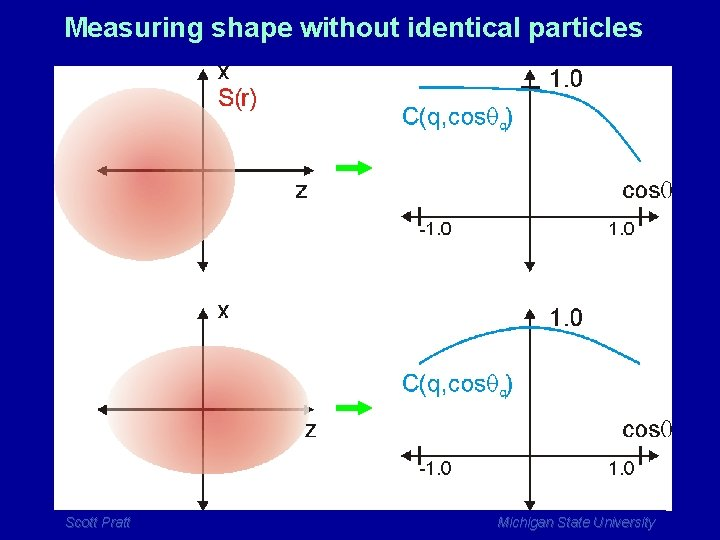Measuring shape without identical particles Scott Pratt Michigan State University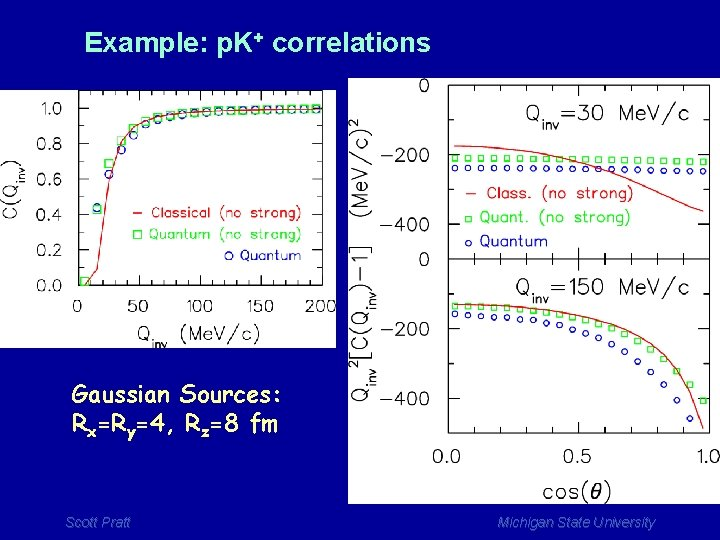Example: p. K+ correlations Gaussian Sources: Rx=Ry=4, Rz=8 fm Scott Pratt Michigan State University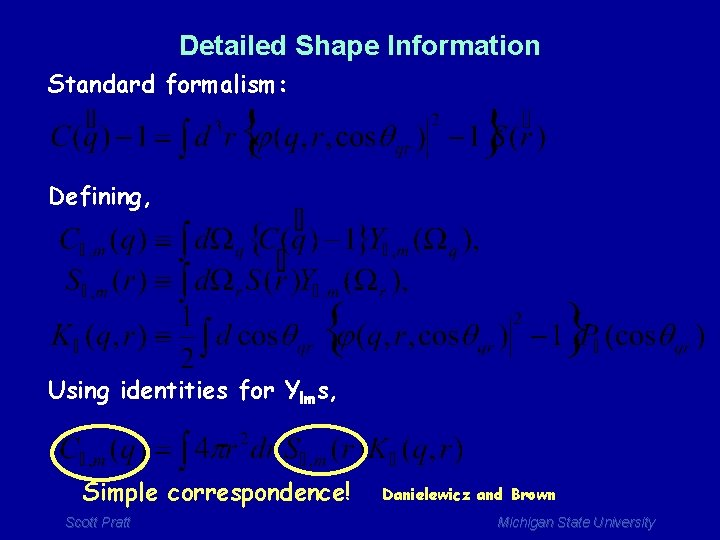Detailed Shape Information Standard formalism: Defining, Using identities for Ylms, Simple correspondence! Scott Pratt Danielewicz and Brown Michigan State University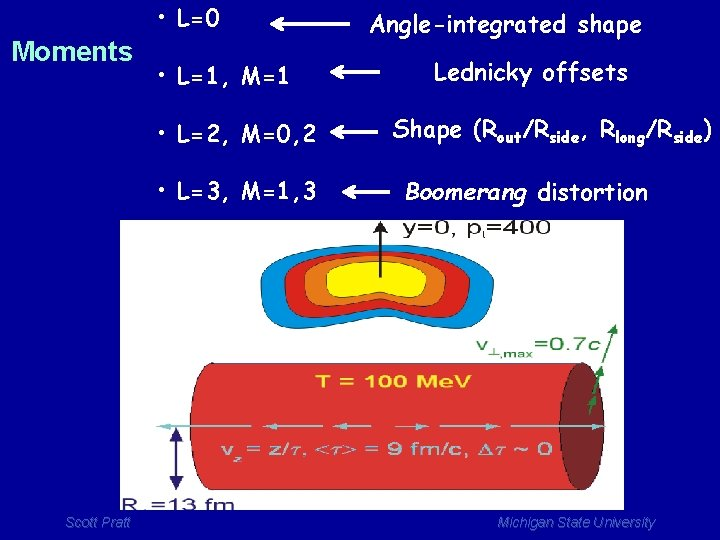• L=0 Moments • L=1, M=1 • L=2, M=0, 2 • L=3, M=1, 3 Scott Pratt Angle-integrated shape Lednicky offsets Shape (Rout/Rside, Rlong/Rside) Boomerang distortion Michigan State University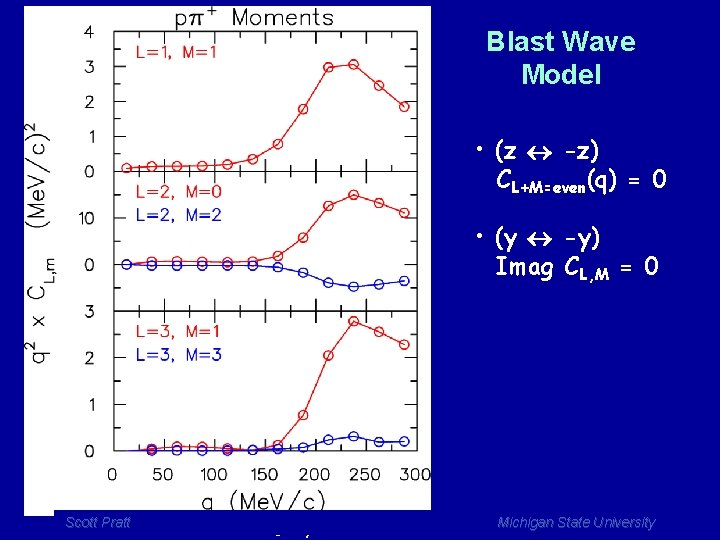Blast Wave Model • (z -z) CL+M=even(q) = 0 • (y -y) Imag CL, M = 0 Scott Pratt S. P. and S. Petriconi, PRC 2003 Michigan State University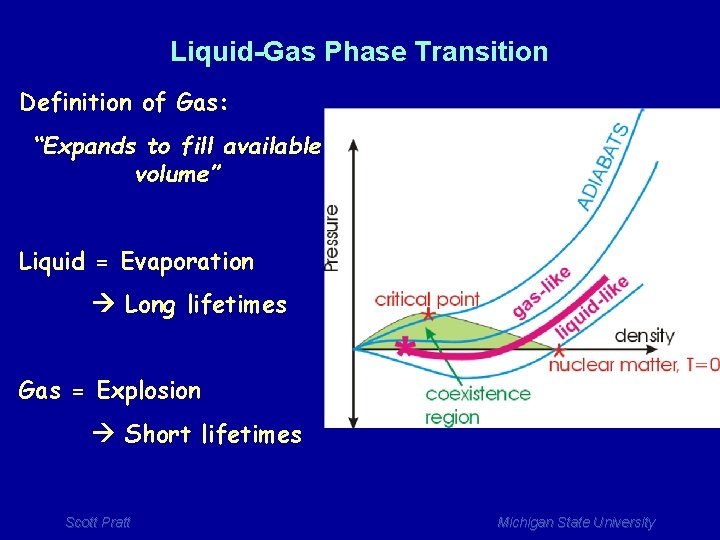Liquid-Gas Phase Transition Definition of Gas: “Expands to fill available volume” Liquid = Evaporation Long lifetimes Gas = Explosion Short lifetimes Scott Pratt Michigan State UniversityChange to Explosive Behavior (GAS) at ~ 50 AMe. V Scott Pratt Michigan State University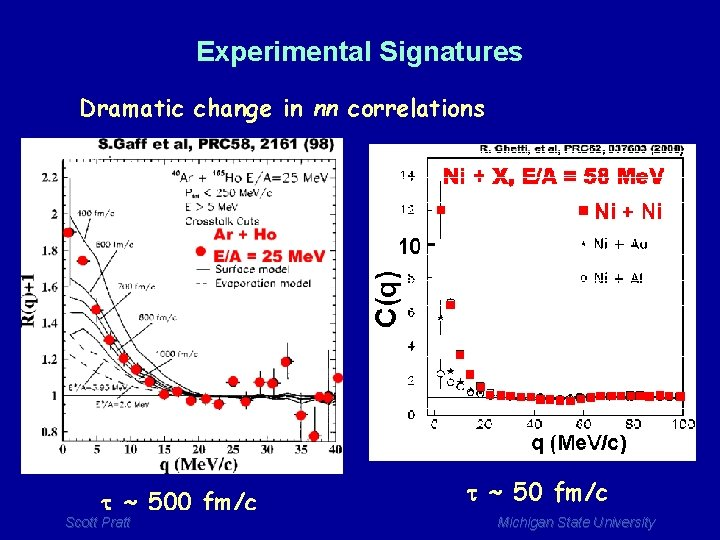Experimental Signatures Dramatic change in nn correlations t ~ 500 fm/c Scott Pratt t ~ 50 fm/c Michigan State University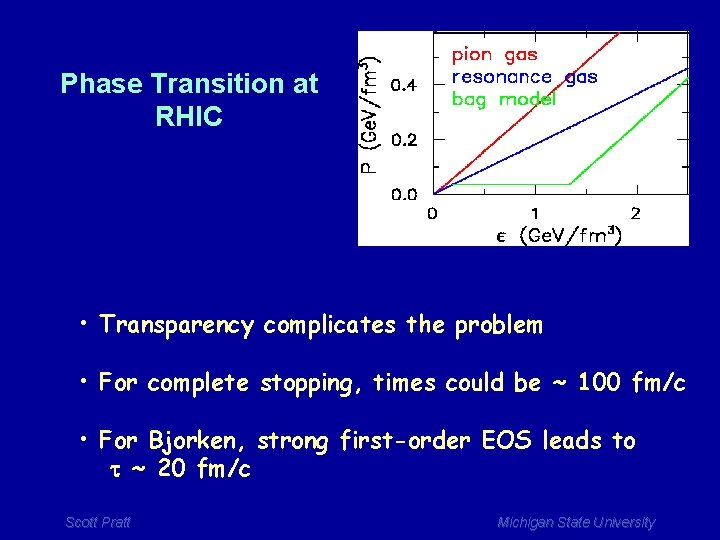Phase Transition at RHIC • Transparency complicates the problem • For complete stopping, times could be ~ 100 fm/c • For Bjorken, strong first-order EOS leads to t ~ 20 fm/c Scott Pratt Michigan State University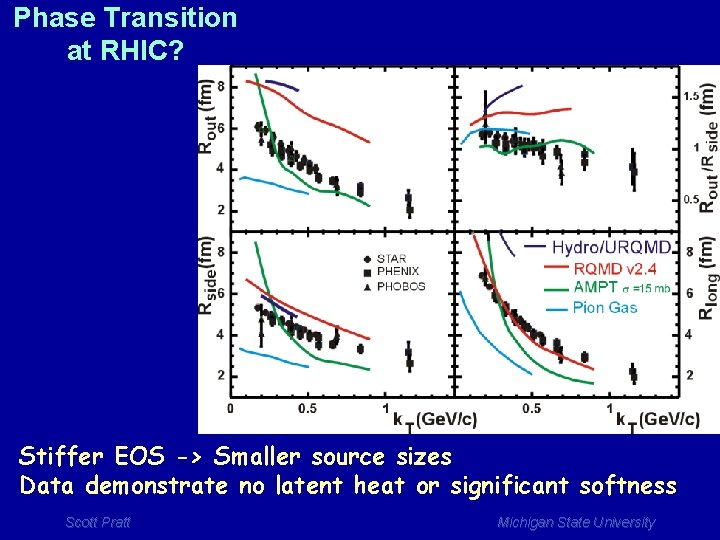Phase Transition at RHIC? Stiffer EOS -> Smaller source sizes Data demonstrate no latent heat or significant softness Scott Pratt Michigan State UniversityTHE HBT PUZZLE AT RHIC To fit data: a) Stiff (but not too stiff) EOS b) Reduce emissivity from surface c) Not that much different than SPS Scott Pratt Michigan State UniversityPhase space density Any method to extract Rinv is sufficient Scott Pratt Michigan State University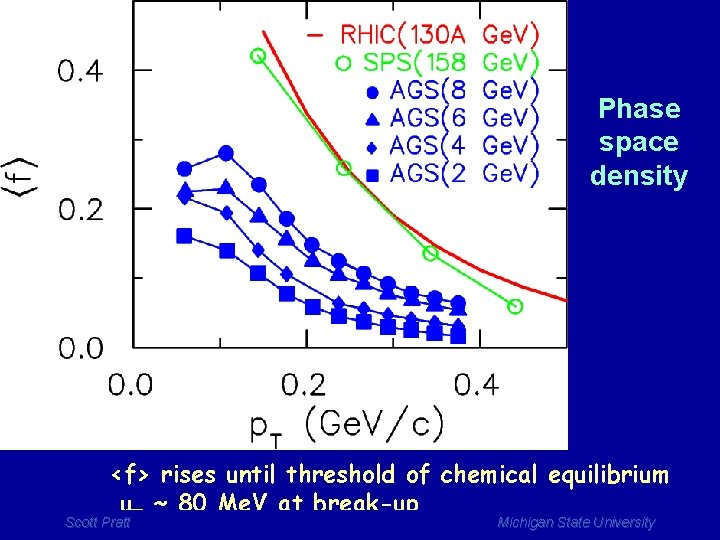Phase space density <f> rises until threshold of chemical equilibrium mp ~ 80 Me. V at break-up Scott Pratt Michigan State University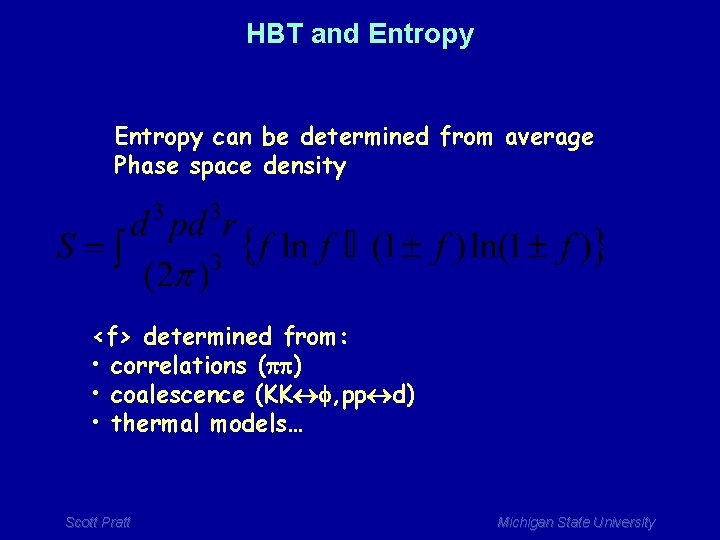HBT and Entropy can be determined from average Phase space density <f> determined from: • correlations (pp) • coalescence (KK f, pp d) • thermal models… Scott Pratt Michigan State University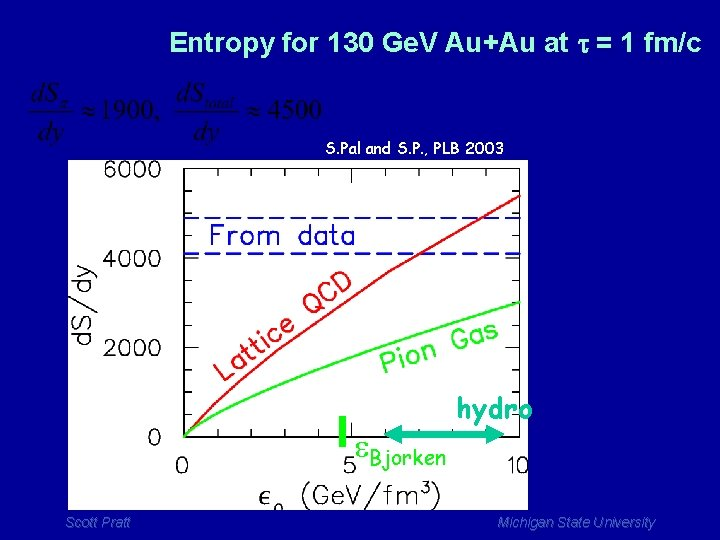Entropy for 130 Ge. V Au+Au at t = 1 fm/c S. Pal and S. P. , PLB 2003 e. Bjorken Scott Pratt hydro Michigan State University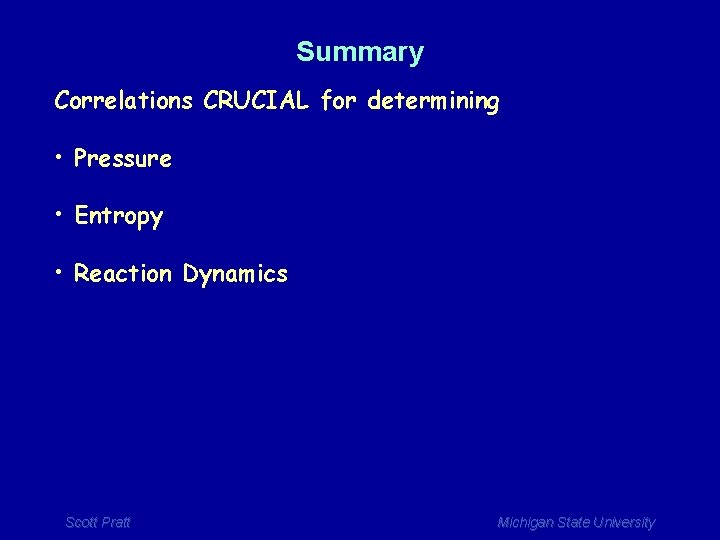Summary Correlations CRUCIAL for determining • Pressure • Entropy • Reaction Dynamics Scott Pratt Michigan State University